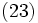# Join of subgroups

## Definition

Suppose$G$ is a group and$H_i$ are subgroups of$G$, where$i \in I$,$I$ an indexing set. The join of the subgroups$H_i$, also called the subgroup generated by the$H_i$s, is defined in the following equivalent way:

• It is the intersection of all subgroups$K \le G$ such that$K$ contains all the$H_i$s
• It is the subgroup generated by the union of the$H_i$s
• It is the set of all elements of$G$ that can be written as a product of elements from the various$H_i$s

The join of finitely many subgroups$H_1, H_2, \ldots, H_n$ is denoted$\langle H_1, H_2, \ldots, H_n \rangle$.

## Related notions

### Union of subgroups

The join of a family of subgroups must contain their union (in fact, it is the subgroup generated by the union). Hence, if the union of a family of subgroups is itself a subgroup, then that equals their join. A particular case of this is when the family of subgroups forms an ascending chain. Another case is where one of the subgroups contains all the others.

Every group is a union of its cyclic subgroups (we take the cyclic subgroup generated by each element). Hence, every group is a join of cyclic subgroups. Further information: every group is a union of cyclic subgroups

A union of two subgroups, neither of which is contained in the other, is not a subgroup. Further information: Union of two subgroups is not a subgroup unless they are comparable

### Product of subgroups

The product of two subgroups$H, K \le G$ is defined as the set of all elements$hk$ where$h \in H, k \in K$. The product depends on the order in which we write the subgroups. In general, the product of two subgroups contains their union and is contained in their join.

In the special case that$HK = KH$, it is a subgroup, and is the same as the join$\langle H, K \rangle$. In such a situation, we say that$H$ and$K$ are permuting subgroups.

## Examples

• In the group$\mathbb{Z}$ of integers, the join of the subgroup$m\mathbb{Z}$ (multiples of$m$) and the subgroup$n\mathbb{Z}$ (multiples of$n$) is the subgroup generated by the gcd of$m$ and$n$. In other words, an element can be written as$ma + nb, a,b \in \mathbb{Z}$ if and only if it is a multiple of the gcd of$m$ and$n$.
• In the symmetric group on three letters, the join of the subgroup generated by the permutation exchanging the first two letters ($(1 2)$) and the permutation exchanging the second and third letter ($(2 3)$) is the whole group. This is an example of a join that is not the same as the union or the product of the subgroups.

## Lattice of subgroups

Further information: Lattice of subgroups

The join of subgroups forms the join operation in the lattice of subgroups. The lattice of subgroups is, as a set, the set of all subgroups, partially ordered by inclusion. The meet operation is intersection of subgroups and the join operation is join of subgroups. The biggest element is the whole group and the smallest element is the trivial subgroup.

## Related subgroup properties and operators

### Join-closed subgroup property

A subgroup property is termed:

• Join-closed if a join of (a nonempty collection of) subgroups, each having the property, also has the property.
• finite-join-closed if a join of a nonempty finite collection of subgroups, each having the property, also has the property.
• strongly join-closed if it is join-closed, and the trivial subgroup has the property (the trivial subgroup arises as the join of the empty collection of subgroups).

### Join-closed group property

• Join-closed group property: A group property is join-closed if, whenever a group can be expressed as a join of subgroups each having the property, then the group also has the property.
• Normal join-closed group property: A group property is normal join-closed if, whenever a group can be expressed as a join of normal subgroups each having the property, then the group also has the property.
• Finite normal join-closed group property: A group property is finite normal join-closed if, whenever a group can be expressed as a join of finitely many normal subgroups each having the property, then the group also has the property. For instance, the property of being a nilpotent group, or the property of being a solvable group.